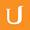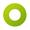## 06-18 All In P Or NP

• 0:00 - 0:06
So our three problems are so closely related that either all of them lie here in NP but not in P
• 0:06 - 0:08
or all of them lie in P.
• 0:08 - 0:11
We don't know which one it is but it can't be the case that,
• 0:11 - 0:15
for example, we have just vertex cover in P and the other two problems here in NP.
• 0:15 - 0:17
And how did we show that in the last unit?
• 0:17 - 0:23
For clique and independent set, we showed that there was a polynomial time algorithm
• 0:23 - 0:28
that could take a graph that was an input to independent set
• 0:28 - 0:32
and transform that into a graph that we can use as an input for clique,
• 0:32 - 0:35
and once we have solved the clique problem on the transformed graph,
• 0:35 - 0:39
that same solution is also the best possible solution to independent set.
• 0:39 - 0:44
So we have a polynomial time algorithm, so the algorithm transforms the input of one problem,
• 0:44 - 0:49
say X, into an input of another problem, Y.
• 0:49 - 0:55
So, for example, we take independent set and transform that graph into an input for clique
• 0:55 - 0:59
and now you'll see one of the advantages of working with decision problems
• 0:59 - 1:04
because the third condition is rather easy to state because we can now simply say that
• 1:04 - 1:09
solving the problem Y on the transformed input yields the same answer
• 1:09 - 1:12
as solving the original problem on the original input.
• 1:12 - 1:16
So these three statements here are the same thing that you have seen in the last unit.
• 1:16 - 1:20
So, we took an input which was a graph to independent set
• 1:20 - 1:25
then transformed it into an input for clique and we found out that if we solve clique on that new input,
• 1:25 - 1:29
then we would get the same answer that we would've gotten
• 1:29 - 1:33
if we had solved independent set directly on the original input.
• 1:33 - 1:36
And of course we also saw that the transformation works in the other way,
• 1:36 - 1:40
so we could transform an input to clique into an input of independent set
• 1:40 - 1:43
and now for a vertex cover and independent set, it was even easier
• 1:43 - 1:48
because we didn't even have to transform the input, so basically the transformation was,
• 1:48 - 1:53
we could keep the input as it is, the only thing is if we're working with the decision problem,
• 1:53 - 1:59
of course, the answer will be a little bit different, so if we have a vertex cover of size k
• 1:59 - 2:03
for a graph with N vertices, then for independent set,
• 2:03 - 2:06
we must ask if we have an independent set of size n-k.
• 2:06 -
But other than that, the transformation here is very easy.
Title:
06-18 All In P Or NP
Team:Udacity
Project:
CS313 - Theoretical Computer Science
Duration:
02:13Amara Bot added a translation

• Amara Bot